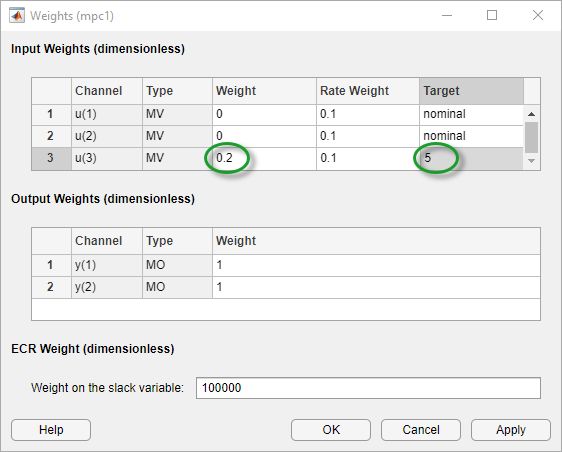## Design MPC Controller for Nonsquare Plant

This topic shows how to configure an MPC controller for a nonsquare plant with unequal numbers of manipulated variables and outputs. Model Predictive Control Toolbox™ software supports plants with an excess of manipulated variables or plant with an excess of outputs.

### More Outputs Than Manipulated Variables

When there are excess outputs, you cannot hold each at a setpoint. In this case, you have two options:

• Specify that certain outputs do not need to be held at setpoints by setting their tuning weights to zero.

The controller does not enforce setpoints on outputs with zero weight, and the outputs are free to vary. If the plant has Ne more outputs than manipulated variables, setting Ne output weights to zero enables the controller to hold the remaining outputs at their setpoints. If any manipulated variables are constrained, one or more output responses can still exhibit steady-state error, depending on the magnitudes of reference and disturbance signals.

Outputs with zero tuning weights can still be useful. If measured, the controller can use the outputs to help estimate the state of the plant. The outputs can also be used as performance indicators or held within an operating region defined by output constraints.

• Enforce setpoints on all outputs by specifying nonzero tuning weights for all of them.

The controller tries to hold all outputs at their respective setpoints. However, due to the limited number of manipulated variables, all output responses exhibit some degree of steady-state error.

You can change the error magnitudes by adjusting the relative values of the output weights. Increasing an output weight decreases the steady-state error in that output at the expense of increased error in the other outputs.

You can configure the output tuning weights at the command line by setting the `Weights.OutputVariables` property of the controller.

To configure output tuning weights in MPC Designer, on the Tuning tab, in the Design section, click Weights to open the Weights dialog box.

In the Output Weights section, specify the Weight for each output variable. For example, if your plant has two manipulated variables and three outputs, you can:

• Set one of the output weights to zero.• Set all the weights to nonzero values. Outputs with higher weights exhibit less steady-state error.### More Manipulated Variables Than Outputs

When there are excessive manipulated variables, the default MPC controller settings allow for error-free output setpoint tracking. However, the manipulated variables values can drift. You can prevent this drift by setting manipulated variable setpoints. If there are Ne excess manipulated variables, and you hold Ne of them at target values for economic or operational reasons, the remaining manipulated variables attain the values required to eliminate output steady-state error.

To configure a manipulated variable setpoint at the command line, use the `ManipulatedVariables.Target` controller property. Then specify an input tuning weight using the controller `Weights.ManipulatedVariables` property.

To define a manipulated variable setpoint in MPC Designer, on the Tuning tab, in the Design section, click Weights.

In the Weights dialog box, in the Input Weights section, specify a nonzero Weight value for the manipulated variable.

Specify a Target value for the manipulated variable.By default, the manipulated variable Target is `nominal`, which means that it tracks the nominal value specified in the controller properties.

Note

Since nominal values apply to all controllers in an MPC Designer session, changing a Nominal Value updates all controllers in the app. The Target value, however, is specific to each individual controller.

The magnitude of the manipulated variable weight indicates how much the input can deviate from its setpoint. However, there is a trade-off between manipulated variable target tracking and output reference tracking. If you want to have better output setpoint tracking performance, use a relatively small input weight. If you want the manipulated variable to stay close to its target value, increase its input weight relative to the output weight.

You can also avoid drift by constraining one or more manipulated variables to a narrow operating region using hard constraints. To define constraints in MPC Designer, on the Tuning tab, in the Design section, click Constraints to open the Constraints dialog box.

In the Input Constraints section, specify Max and Min constraints values.